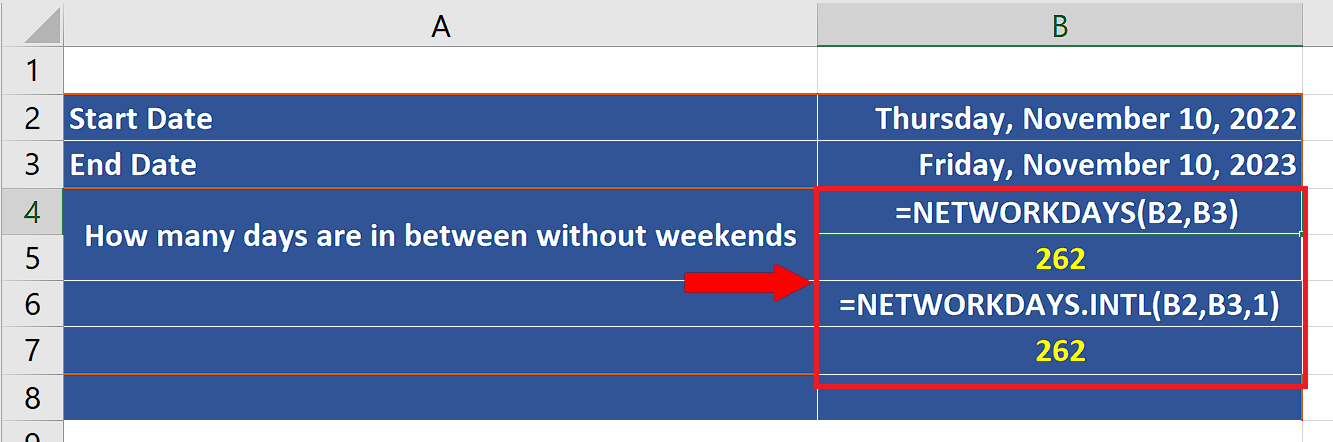# How to find number of days in a year without weekends in Excel

In today’s tutorial we are going to learn how to find the number of days in a year excluding the weekends. Excel has a very simple formula i.e. NETWORKDAYS and NETWORKDAYS.INTL to perform this calculation. Let’s learn about the syntax of both  formulas first.

## Syntax of the formulas

Lets’ learn about the formulas first and then implement both of these to find the number of days.

NETWORKDAYS(start_date, end_date, [holidays])

start_date:

This refers to the start date in the calculation. It can’t be later than the end date.

end_date:

This refers to the end date in the calculation. It can’t be earlier than the start date.

[holidays]:

This is an optional parameter in this formula and it can be a list of official holidays other than the weekends which must be provided manually to the formula in the form of dates. Dates should be entered by using the DATE function, or as results of other formulas or functions. For example, use DATE(2022,11,23) for the 23rd day of Nov, 2022. Problems can occur if dates are entered as text.

The second formula is;

NETWORKDAYS.INTL(start_date, end_date, [weekend], [holidays])

start_date:

This refers to the start date in the calculation. It can’t be later than the end date.

end_date:

This refers to the end date in the calculation. It can’t be earlier than the start date.

[weekend]:

This parameter is an optional one but it is a very important one if properly used. By using this we can nominate any day/days of the week as weekend. The options are entered in the form of numeric data.

[holidays]:

This is an optional parameter in this formula and it can be a list of official holidays other than the weekends which must be provided manually to the formula in the form of dates. Dates should be entered by using the DATE function, or as results of other formulas or functions. For example, use DATE(2022,11,23) for the 23rd day of Nov, 2022. Problems can occur if dates are entered as text.

Now let’s implement the formula and find out the number of days in a year.If you are a project manager then working with dates and days is an inevitable requirement. Microsoft Excel provides very useful methods to work with days and dates for the ease of project managers.

### Step 1 – Implement the formula to calculate days without weekends in a year– Write start and end date in two different cells.

– Setup both formulas as shown in the figure below and we’ll see that we get the same result in both formulas. It is due to the fact that the first formula assumes that Saturdays and Sundays are the weekends and in the second formula we chose the weekend option 1 which also tells the formula to assume that Saturdays and Sundays are the weekends.